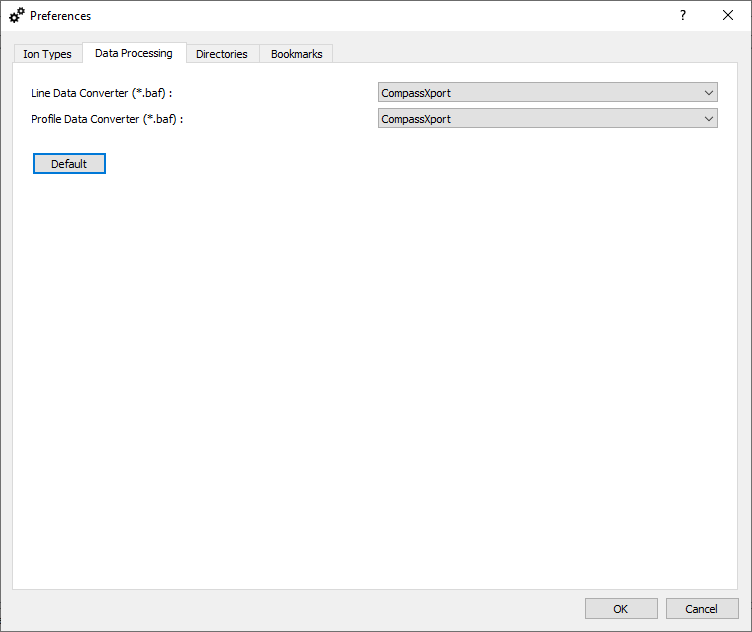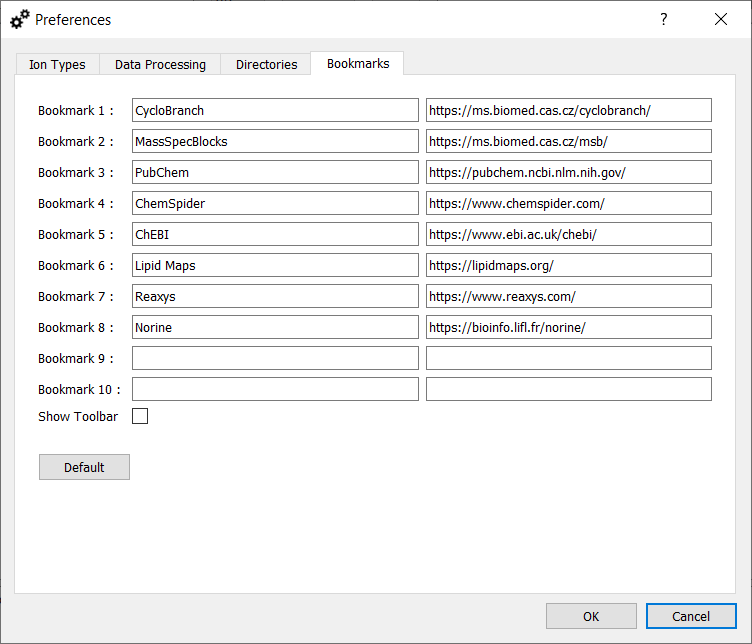CycloBranch
Preferences

# Preferences Dialog

The preferences dialog is opened using the command "File -> Preferences". Use F1 to open this help.

In this dialog, you can define global preferences of the application, which are stored in the "CycloBranch.ini" file. The preferences dialog has the following tabs:

## Ion TypesIon Types.

In this tab you can define a list of custom ions, which are shown in the Settings dialog if MS, LC-MS, and MSI data is processed. Buttons:

• 'Add' - add a new entry at the end of the list
• 'Edit' - edit selected item
• 'Remove' - remove selected item
• 'Default' - clear the current list and define a default list of ions (see below)

The dialog 'Define Ion Type' includes the following rows:

• 'Name' - a unique name of ion, e.g., [M+H]+.
• 'Formula' - the formula 'H+' determines the difference between a neutral molecule and the ion. The symbol '+' has a special meaning and it determines that a mass of electron is subtracted. For example, if you would like to define the ion [M-H]-, the correct syntax is 'H-1+-1', where '+-1' means that the mass of electron is added.
• 'Mode' - define mode in which the ion type can be used - positive or negative.
• 'M' - define the number n of connected molecules in [nM+H]+, e.g., n=2 in [2M+Fe-3H+Na]+.

The default list of ion types includes the following items:

• [M+H]+
• [M+Na]+
• [M+K]+
• [M-H]-
• [M+Na-2H]-
• [M+K-2H]-
• [M+Fe-2H]+
• [M+Fe-3H+Na]+
• [M+Fe-3H+K]+
• [2M+Fe-2H]+
• [2M+Fe-3H+Na]+
• [2M+Fe-3H+K]+
• [3M+Fe-2H]+
• [3M+Fe-3H+Na]+
• [3M+Fe-3H+K]+
• [3M+2Fe-5H]+
• [3M+2Fe-6H+Na]+
• [3M+2Fe-6H+K]+
• [M+Fe-4H]-
• [2M+Fe-4H]-
• [3M+Fe-4H]-
• [3M+2Fe-7H]-
• [M+NH4]+
• [M+Cl]-
• [M]+
• [M]-
• [M+Li]+
• [M+Mg-H]+
• [M+Mg-2H+Na]+
• [M+Mg-2H+K]+
• [M+Mg-3H]-
• [M+Al-2H]+
• [M+Al-3H+Na]+
• [M+Al-3H+K]+
• [M+Al-4H]-
• [M+Si-3H]+
• [M+Si-4H+Na]+
• [M+Si-4H+K]+
• [M+Si-5H]-
• [M+Ca-H]+
• [M+Ca-2H+Na]+
• [M+Ca-2H+K]+
• [M+Ca-3H]-
• [M+Sc-2H]+
• [M+Sc-3H+Na]+
• [M+Sc-3H+K]+
• [M+Sc-4H]-
• [M+Cr-2H]+
• [M+Cr-3H+Na]+
• [M+Cr-3H+K]+
• [M+Cr-4H]-
• [M+Mn-H]+
• [M+Mn-2H+Na]+
• [M+Mn-2H+K]+
• [M+Mn-3H]-
• [M+Co-H]+
• [M+Co-2H+Na]+
• [M+Co-2H+K]+
• [M+Co-3H]-
• [M+Ni-H]+
• [M+Ni-2H+Na]+
• [M+Ni-2H+K]+
• [M+Ni-3H]-
• [M+Cu-H]+
• [M+Cu-2H+Na]+
• [M+Cu-2H+K]+
• [M+Cu-3H]-
• [M+Zn-H]+
• [M+Zn-2H+Na]+
• [M+Zn-2H+K]+
• [M+Zn-3H]-
• [M+Ga-2H]+
• [M+Ga-3H+Na]+
• [M+Ga-3H+K]+
• [M+Ga-4H]-

## Data ProcessingData processing options.

Define a tool used for extraction of line/profile mass spectra from *.baf files. The corresponding tool (CompassXport 3.0.13.1 or CompassXtract 3.2.201 (64-bit)) must be installed separately.

## DirectoriesDefault Directories.

In this tab, you can define the default folders for various types of input/output files.

## BookmarksDefault bookmarks.

A custom list of up to 10 bookmarks can be created. The bookmarks can then be opened in an external web browser using CTRL + 1, CTRL + 2, ..., CTRL + 9, and CTRL + 0. Select "Show Toolbar" to display a toolbar with bookmarks in the main window of the application.